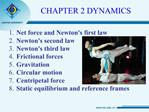DownloadDownload PresentationNet force and Newtons first law Newtons second law Newtons third law Frictional forces Gravitation Circular moti

# Net force and Newtons first law Newtons second law Newtons third law Frictional forces Gravitation Circular moti

Télécharger la présentation## Net force and Newtons first law Newtons second law Newtons third law Frictional forces Gravitation Circular moti

- - - - - - - - - - - - - - - - - - - - - - - - - - - E N D - - - - - - - - - - - - - - - - - - - - - - - - - - -
##### Presentation Transcript

2. Who are they??? Universal Laws of Classical Mechanics a = v2/R

3. What is the difference between Kinematics and Dynamics ?

4. Part 1

5. Review: Vector sum of Net force Net force: System of many vectors act to one mass point O. Example:

6. Learning Check

7. Newton�s First Law @ The motion of an object does not change unless it is acted upon by a net force. * If v = 0, it remains at rest. * If v = C, it till move at that value. @ Another way to say the same thing: No net force ? call freely moving object Velocity is constant or zero ? acceleration is zero

9. Learning check Suppose you are on an airplane travelling at constant velocity with a speed of 500 miles per hour (roughly 200 m/s) If you throw a ball straight up, does it return to you?

10. Valuable Solution

12. Question 1 An airplane flying: Many forces act on the plane, including weight (gravity), drag (air resistance), the thrust of the engine, and the lift of the wings. At some point during its trip the velocity of the plane is measured to be constant (which means its altitude is also constant). At this time, the total force on the plane: 1. is pointing upward 2. is pointing downward 3. is pointing forward 4. is pointing backward 5. is zero

14. Contact and Field Forces

15. MCQ Test Forces that appear in contact A. Friction B. Electron acts to another electron C. Gravity (you and the Earth) D. Two mobiphones at distance of 5m.

18. Mass or Inertia Inertia is the tendency of an object to remain at rest or in motion with constant speed along a straight line. Mass (m) is the quantitative measure of inertia. Mass is the property of an object that measures how hard it is to change its motion. Units: [M] = kg

19. Learning check Look at all people in this ficture, who has smallest inertia ?

21. Mass vs. Weight Mass is an intrinsic property of an object. A rock has same mass whether it is on the moon or on Earth Weight is the force exerted on an object by gravity. This is different depending upon the strength of the gravitational force. On the surface of Earth, gravitational force is constant so we can easily convert from mass to weight.

22. Galileo�s Profound Contributions to Physics Include: Principle of inertia

23. Part 2 Newton�s second law

25. Learning Check

26. How Does a Car Move? Each arrow represents a force. Your car is accelerating forward, meaning there is a net force in that direction. Identical concept for walking (or running).

28. Which has more momentum? A bullet, mass = 100 grams, speed = 1000m/s A car, mass=1000kg, speed = 10m/s

30. Newton�s Second Law This law tells us how motion changes when a net force is applied. acceleration = (net force)/mass Stimulus is Force (cause) Resistance is mass (control rod) Response is acceleration (effect)Stimulus is Force (cause) Resistance is mass (control rod) Response is acceleration (effect)

31. Newton�s Second Law Units: Prove: [F] = [M] [a] [F] = kg m/s2 1 Newton (N) ? 1 kg m/s2 A scaler equation: Fnet,x = Max Fnet,y = May

33. Part 3 Newton �s third law

36. Newton�s 2. and 3. Laws Suppose you are an astronaut in outer space giving a brief push to a spacecraft whose mass is bigger than your own Compare the magnitude of the force you exert on the spacecraft, FS, to the magnitude of the force exerted by the spacecraft on you, FA, while you are pushing: 1.FA = FS 2. FA > FS 3. FA < FS

37. Exercise: Action-Reaction Suppose a tennis ball (m= 0.1 kg) moving at a velocity v = 40 m/sec collides head-on with a truck (M = 500 kg) which is moving with velocity V = 10 m/sec. During the collision, the tennis ball exerts a force on the truck which is smaller than the force which the truck exerts on the tennis ball. TRUE or FALSE ? The tennis ball will suffer a larger acceleration during the collision than will the truck. TRUE or FALSE ? Suppose the tennis ball bounces away from the truck after the collision. How fast is the truck moving after the collision? < 10 m/sec = 10 m/sec > 10 m/sec ?

41. Short thesis Do the thesis about 1- Application of Crane truck 2- Combine harvester machine 3- Centrifuge machine 4- Automatic teller machine; ATM 5- Meat grinder machine

42. Please Make friend with DVD experiments